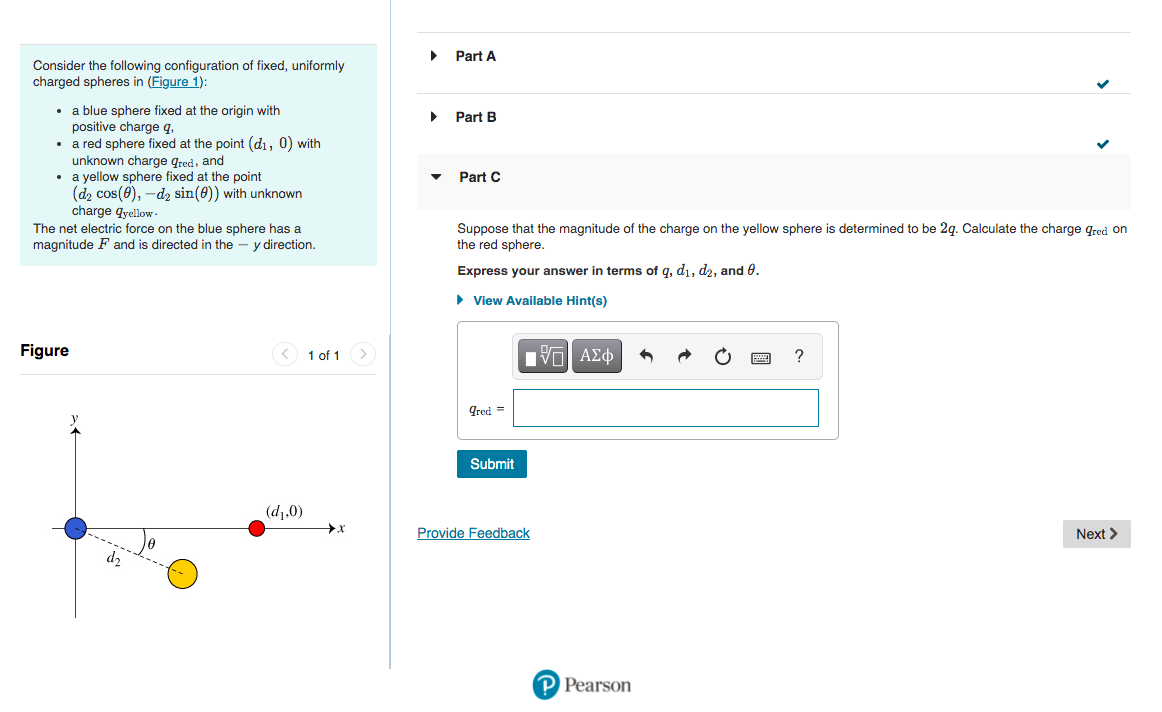# Question Solved1 Answer Part A Part B Consider the following configuration of fixed, uniformly charged spheres in (Figure 1): • a blue sphere fixed at the origin with positive charge q, a red sphere fixed at the point (di, 0) with unknown charge Gred, and • a yellow sphere fixed at the point (d, cos((), -d, sin(()) with unknown charge qyellow The net electric force on the blue sphere has a magnitude F and is directed in the - y direction. Part Suppose that the magnitude of the charge on the yellow sphere is determined to be 27. Calculate the charge qred on the red sphere. Express your answer in terms of q, di, dz, and . View Available Hint(s) Figure 1 of 1 EVO AEO ? Pred - Submit (0,0) Provide Feedback Next > PPearsonTranscribed Image Text: Transcribed image text: Part A Part B Consider the following configuration of fixed, uniformly charged spheres in (Figure 1): • a blue sphere fixed at the origin with positive charge q, a red sphere fixed at the point (di, 0) with unknown charge Gred, and • a yellow sphere fixed at the point (d, cos((), -d, sin(()) with unknown charge qyellow The net electric force on the blue sphere has a magnitude F and is directed in the - y direction. Part Suppose that the magnitude of the charge on the yellow sphere is determined to be 27. Calculate the charge qred on the red sphere. Express your answer in terms of q, di, dz, and . View Available Hint(s) Figure 1 of 1 EVO AEO ? Pred - Submit (0,0) Provide Feedback Next > PPearson
More
Transcribed Image Text: Transcribed image text: Part A Part B Consider the following configuration of fixed, uniformly charged spheres in (Figure 1): • a blue sphere fixed at the origin with positive charge q, a red sphere fixed at the point (di, 0) with unknown charge Gred, and • a yellow sphere fixed at the point (d, cos((), -d, sin(()) with unknown charge qyellow The net electric force on the blue sphere has a magnitude F and is directed in the - y direction. Part Suppose that the magnitude of the charge on the yellow sphere is determined to be 27. Calculate the charge qred on the red sphere. Express your answer in terms of q, di, dz, and . View Available Hint(s) Figure 1 of 1 EVO AEO ? Pred - Submit (0,0) Provide Feedback Next > PPearson